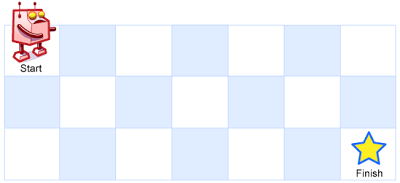# LeetCode 91. Decode Ways

## Description

Decode Ways

A message containing letters from A-Z is being encoded to numbers using the following mapping:

``````'A' -> 1
'B' -> 2
...
'Z' -> 26
``````

Given a non-empty string containing only digits, determine the total number of ways to decode it.

Example 1:

``````Input: "12"
Output: 2
Explanation: It could be decoded as "AB" (1 2) or "L" (12).
``````

Example 2:

``````Input: "226"
Output: 3
Explanation: It could be decoded as "BZ" (2 26), "VF" (22 6), or "BBF" (2 2 6).
``````

# LeetCode 87. Scramble String

## Description

Scramble String

Given a string s1, we may represent it as a binary tree by partitioning it to two non-empty substrings recursively.

Below is one possible representation of s1 = `"great"`:

``````    great
/    \
gr    eat
/ \    /  \
g   r  e   at
/ \
a   t
``````

To scramble the string, we may choose any non-leaf node and swap its two children.

# LeetCode 85. Maximal Rectangle

## Description

Maximal Rectangle

Given a 2D binary matrix filled with 0's and 1's, find the largest rectangle containing only 1's and return its area.

Example:

``````Input:
[
["1","0","1","0","0"],
["1","0","1","1","1"],
["1","1","1","1","1"],
["1","0","0","1","0"]
]
Output: 6
``````

# LeetCode 72. Edit Distance

## Description

Edit Distance

Given two words word1 and word2, find the minimum number of operations required to convert word1 to word2.

You have the following 3 operations permitted on a word:

1. Insert a character
2. Delete a character
3. Replace a character

Example 1:

Input: word1 = “horse”, word2 = “ros” Output: 3 Explanation: horse -> rorse (replace ‘h’ with ‘r’) rorse -> rose (remove ‘r’) rose -> ros (remove ‘e’)

``````>
> **Example 2:**
>
> ```
Input: word1 = "intention", word2 = "execution"
Output: 5
Explanation:
intention -> inention (remove 't')
inention -> enention (replace 'i' with 'e')
enention -> exention (replace 'n' with 'x')
exention -> exection (replace 'n' with 'c')
exection -> execution (insert 'u')
``````

# LeetCode 70. Climbing Stairs

## Description

Climbing Stairs

You are climbing a stair case. It takes n steps to reach to the top.

Each time you can either climb 1 or 2 steps. In how many distinct ways can you climb to the top?

Note: Given n will be a positive integer.

Example 1:

``````Input: 2
Output: 2
Explanation: There are two ways to climb to the top.
1. 1 step + 1 step
2. 2 steps
``````

Example 2:

``````Input: 3
Output: 3
Explanation: There are three ways to climb to the top.
1. 1 step + 1 step + 1 step
2. 1 step + 2 steps
3. 2 steps + 1 step
``````

# LeetCode 64. Minimum Path Sum

## Description

Minimum Path Sum

Given a m x n grid filled with non-negative numbers, find a path from top left to bottom right which minimizes the sum of all numbers along its path.

Note: You can only move either down or right at any point in time.

Example:

``````Input:
[
[1,3,1],
[1,5,1],
[4,2,1]
]
Output: 7
Explanation: Because the path 1→3→1→1→1 minimizes the sum.
``````

# LeetCode 63. Unique Paths II

## Description

Unique Paths II

A robot is located at the top-left corner of a m x n grid (marked ‘Start’ in the diagram below).

The robot can only move either down or right at any point in time. The robot is trying to reach the bottom-right corner of the grid (marked ‘Finish’ in the diagram below).

Now consider if some obstacles are added to the grids. How many unique paths would there be?An obstacle and empty space is marked as 1 and 0 respectively in the grid.

Note: m and n will be at most 100.

Example 1:

``````Input:
[
[0,0,0],
[0,1,0],
[0,0,0]
]
Output: 2
Explanation:
There is one obstacle in the middle of the 3x3 grid above.
There are two ways to reach the bottom-right corner:
1. Right -> Right -> Down -> Down
2. Down -> Down -> Right -> Right
``````

# LeetCode 62. Unique Paths

## Description

Unique Paths

A robot is located at the top-left corner of a m x n grid (marked ‘Start’ in the diagram below).

The robot can only move either down or right at any point in time. The robot is trying to reach the bottom-right corner of the grid (marked ‘Finish’ in the diagram below).

How many possible unique paths are there?Programer

ChengDu·China